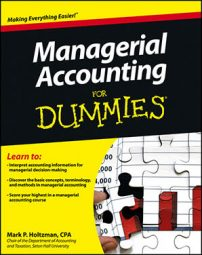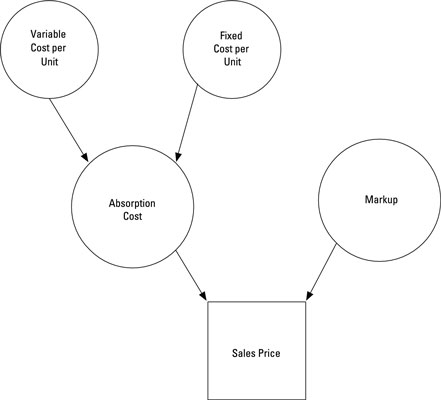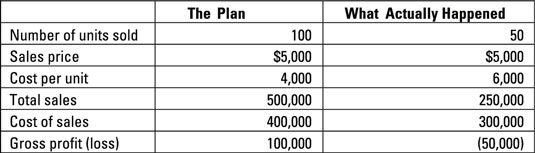##### Managerial Accounting For DummiesMany retailers and manufacturers set their prices at cost-plus by adding a fixed markup to their absorption cost. Cost-plus pricing ensures that prices are high enough to meet profit goals. The figure illustrates how cost-plus pricing computes the sales price by adding markup to a product’s fixed and variable costs.## How to compute fixed markups

To figure out the markup for cost-plus pricing, divide total desired profit by the number of units produced.

For example, suppose that Saint Company wants to earn \$100,000 on the production of 100 Model 51 Robots:

Desired profit / Units produced = Markup
\$100,000 / 100 = Markup
\$1,000 = Markup

Dividing the desired profit by units produced results in a planned markup of \$1,000 per unit. To set the price, add this planned markup to the cost. Assume that Saint’s cost to produce each robot is \$4,000:

Cost + Markup = Sales price
\$4,000 + \$1,000 = Sales price
\$5,000 = Sales price

If Saint Company wants to earn a total of \$100,000, it should set the price at \$5,000 per unit.

## How to set a cost-plus percentage

Because companies often sell many different products at different prices, they commonly use a cost-plus percentage or percentage markup on cost that applies to all their products. To figure this percentage, you divide the markup, in dollars, by the expected sales price. Then, to determine the products’ sales prices, you apply this percentage to all products, or to different categories of products.

For example, Saint Company’s Model 51 Robot has a \$1,000 markup on a sales price of \$5,000.

Markup / Sales price = Cost-plus percentage
\$1,000 / \$5,000 = Cost-plus percentage
20% = Cost-plus percentage

Here, Saint earns a 20-percent cost-plus percentage. The company can then apply the same cost-plus percentage to set the prices of other products. For example, another robot, Model 6, costs Saint Company \$6,500 to produce. The markup on this robot amounts to \$1,300 (\$6,500 x 20 percent), pricing it at \$7,800 (\$6,500 + \$1,300).

## Problems with cost-plus pricing

Cost-plus pricing works because it’s easy to use. However, it carries a few drawbacks. First, it ignores market factors. Just because you like to mark up your merchandise 20 percent doesn’t necessarily mean your customers are willing to pay this price or that your competitors will cooperate with you by setting their prices even higher.

Second, because cost-plus pricing relies on absorption costing, it treats fixed costs as though they were variable. Saint Company wants to sell 100 Model 51 Robots, which means it can distribute its fixed costs over 100 units.

However, the fixed costs remain the same regardless of how many units Saint actually sells; if the company sells only 50 robots, the fixed costs are spread over fewer units (50 robots rather than 100), and the cost per unit rises. Here, if production drops to 50 robots, then the cost per unit increases to \$6,000 per unit. This change puts Saint into a tight bind.Saint originally assumes it will make and sell 100 units. Based on this assumption, it projects an average cost of \$4,000 per unit, a markup of \$1,000, and a sales price of \$5,000. However, if Saint makes and sells only 50 units, the average cost balloons to \$6,000 per unit. Stuck with a sales price of \$5,000, Saint loses \$50,000.

Look on the bright side: If your sales volume is higher than you expected, then it will have a disproportionately positive effect on income — delivering profits way beyond your wildest dreams.